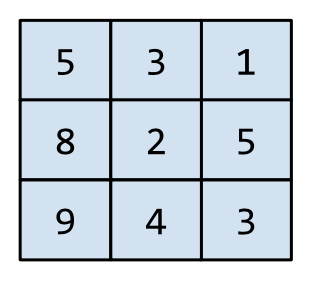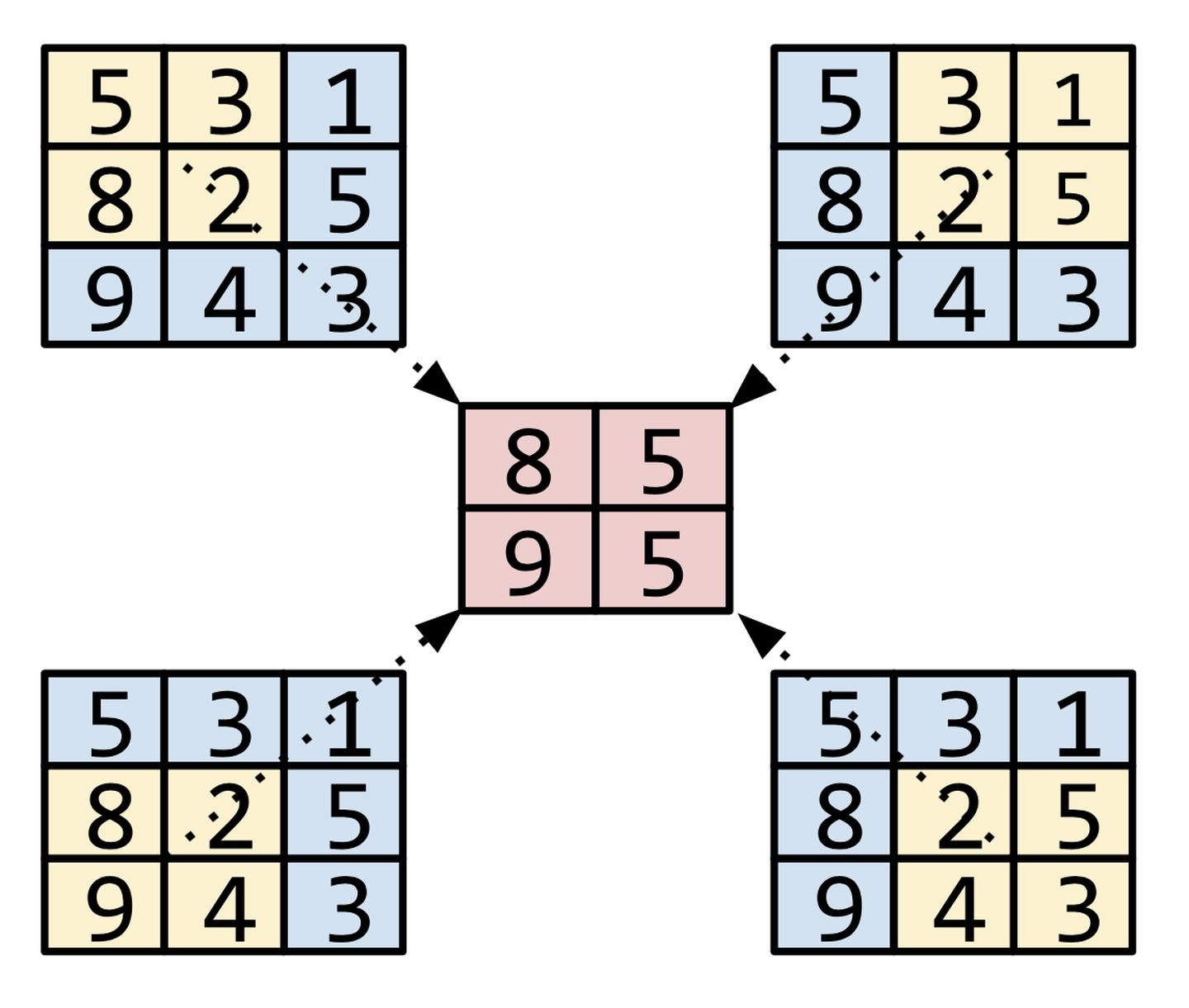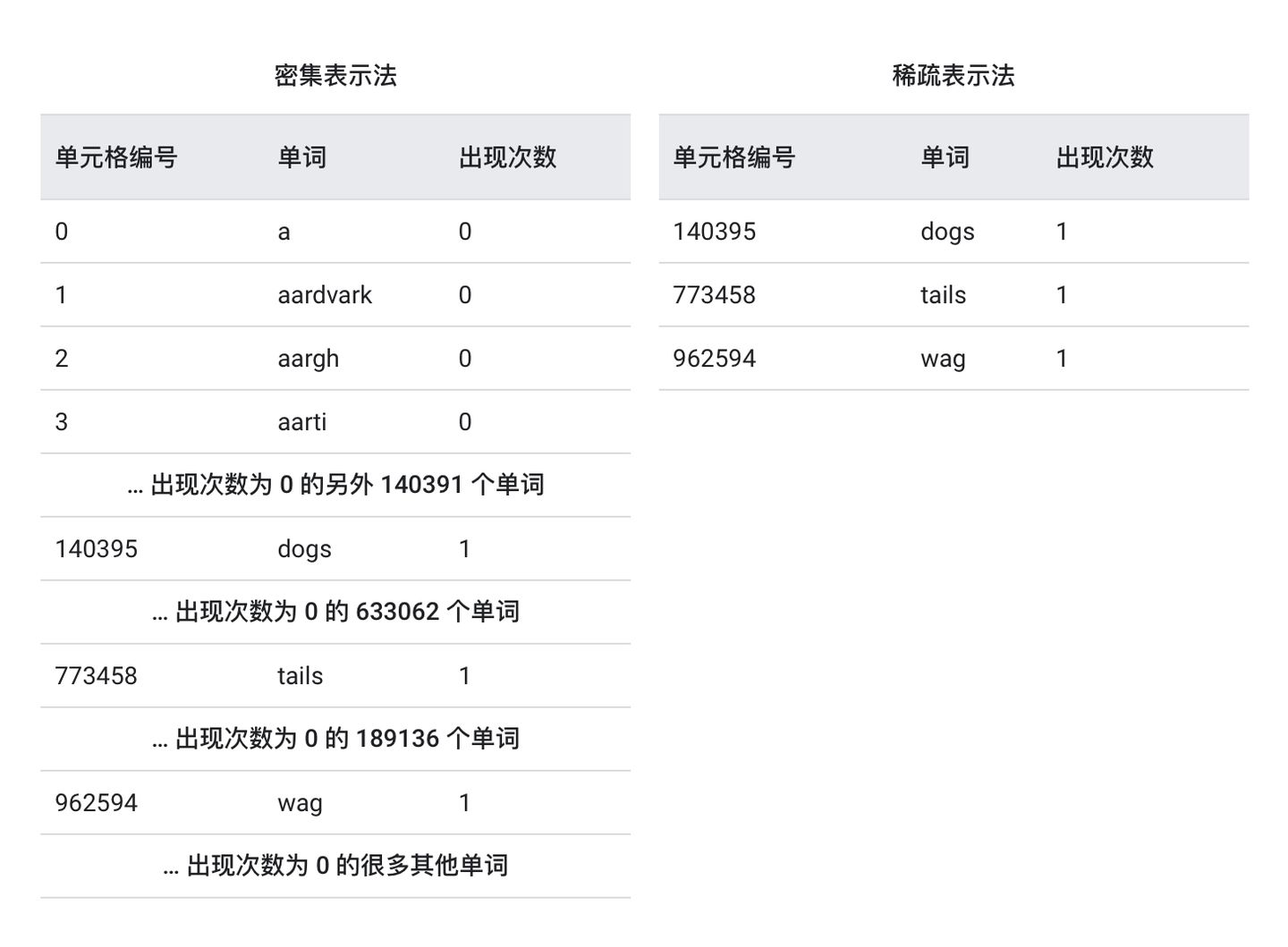﻿• 价格透明
• 信息保密
• 进度掌控
• 售后无忧# 超全汇总！机器学习常用术语词汇表——下篇（建议收藏）

## N

• NaN 陷阱 (NaN trap)

NaN 是“非数字”的缩写。

• 负类别 (negative class)

• 神经网络 (neural network)

• 神经元 (neuron)

• 节点 (node)

• 隐藏层中的神经元。
• TensorFlow 图中的操作。

• 标准化 (normalization)

• 数值数据 (numerical data)

• Numpy

## O

• 目标 (objective)

• 离线推断 (offline inference)

• 独热编码 (one-hot encoding)

• 一个元素设为 1。
• 所有其他元素均设为 0。

• 单样本学习（one-shot learning，通常用于对象分类）

• 一对多 (one-vs.-all)

• 动物和非动物
• 蔬菜和非蔬菜
• 矿物和非矿物

• 在线推断 (online inference)

• 操作 (op, Operation)

TensorFlow 图中的节点。在 TensorFlow 中，任何创建、操纵或销毁张量的过程都属于操作。例如，矩阵相乘就是一种操作，该操作以两个张量作为输入，并生成一个张量作为输出。

• 优化器 (optimizer)

• 动量 (Momentum)
• 稀疏性/正则化 (Ftrl)
• 更复杂的数学方法（Proximal，等等）

• 离群值 (outlier)

• 绝对值很高的权重。
• 与实际值相差很大的预测值。
• 值比平均值高大约 3 个标准偏差的输入数据。

• 输出层 (output layer)

• 过拟合 (overfitting)

## P

• Pandas

• 参数 (parameter)

• 参数服务器 (PS, Parameter Server)

• 参数更新 (parameter update)

• 偏导数 (partial derivative)

• 划分策略 (partitioning strategy)

• 性能 (performance)

• 在软件工程中的传统含义。即：相应软件的运行速度有多快（或有多高效）？
• 在机器学习中的含义。在机器学习领域，性能旨在回答以下问题：相应模型的准确度有多高？即模型在预测方面的表现有多好？

• 困惑度 (perplexity)

$$P= 2^{-\text{cross entropy}}$$

• 流水线 (pipeline)

## 池化 (pooling)• 正类别 (positive class)

• 精确率 (precision)

• 预测 (prediction)

• 预测偏差 (prediction bias)

• 预训练模型 (pre-trained model)

• 先验信念 (prior belief)

• 队列 (queue)

## R

• 等级 (rank)

• 张量中的维数。例如，标量等级为 0，向量等级为 1，矩阵等级为 2。
• 在将类别从最高到最低进行排序的机器学习问题中，类别的顺序位置。例如，行为排序系统可以将狗狗的奖励从最高（牛排）到最低（枯萎的羽衣甘蓝）进行排序。

• 评分者 (rater)

• 召回率 (recall)

$$\text{召回率} = \frac{\text{正例数}} {\text{正例数} + \text{假负例数}}$$

• 修正线性单元 (ReLU, Rectified Linear Unit)

• 如果输入为负数或 0，则输出 0。
• 如果输入为正数，则输出等于输入。

• 回归模型 (regression model)

• 正则化 (regularization)

• 丢弃正则化
• 早停法（这不是正式的正则化方法，但可以有效限制过拟合）

• 正则化率 (regularization rate)

• 表示法 (representation)

• 受试者工作特征曲线（receiver operating characteristic，简称 ROC 曲线）

• 根目录 (root directory)

• 均方根误差 (RMSE, Root Mean Squared Error)

• 旋转不变性 (rotational invariance)

## S

• SavedModel

• Saver

• 缩放 (scaling)

• scikit-learn

• 半监督式学习 (semi-supervised learning)

• 序列模型 (sequence model)

• 会话 (tf.session)

• S 型函数 (sigmoid function)

$$y = \frac{1}{1 + e^{-\sigma}}$$

$$\sigma = b + w_1x_1 + w_2x_2 + … w_nx_n$$

• 大小不变性 (size invariance)

• softmax

• 稀疏特征 (sparse feature)

• 稀疏表示法 (sparse representation)

• 要采用密集表示法来表示此句子，则必须为所有一百万个单元格设置一个整数，然后在大部分单元格中放入 0，在少数单元格中放入一个非常小的整数。
• 要采用稀疏表示法来表示此句子，则仅存储象征句子中实际存在的单词的单元格。因此，如果句子只包含 20 个独一无二的单词，那么该句子的稀疏表示法将仅在 20 个单元格中存储一个整数。• 稀疏性 (sparsity)

$${\text{稀疏性}} = \frac{\text{98}} {\text{100}} = {\text{0.98}}$$

• 空间池化 (spatial pooling)

• 平方合页损失函数 (squared hinge loss)

• 平方损失函数 (squared loss)

• 静态模型 (static model)

• 平稳性 (stationarity)

• 步 (step)

• 步长 (step size)

• 随机梯度下降法 (SGD, stochastic gradient descent)

• 结构风险最小化 (SRM, structural risk minimization)

• 期望构建最具预测性的模型（例如损失最低）。
• 期望使模型尽可能简单（例如强大的正则化）。

• 步长 (stride)• 下采样 (subsampling)

• 总结 (summary)

• 监督式机器学习 (supervised machine learning)

• 合成特征 (synthetic feature)

• 对连续特征进行分桶，以分为多个区间分箱。
• 将一个特征值与其他特征值或其本身相乘（或相除）。
• 创建一个特征组合。

## T

• 目标 (target)

• 时态数据 (temporal data)

• 张量 (Tensor)

TensorFlow 程序中的主要数据结构。张量是 N 维（其中 N 可能非常大）数据结构，最常见的是标量、向量或矩阵。张量的元素可以包含整数值、浮点值或字符串值。

• 张量处理单元 (TPU, Tensor Processing Unit)

• 张量等级 (Tensor rank)

• 张量形状 (Tensor shape)

• 张量大小 (Tensor size)

• TensorBoard

• TensorFlow

• TensorFlow Playground

• TensorFlow Serving

• 测试集 (test set)

• tf.Example

• 时间序列分析 (time series analysis)

• 训练 (training)

• 训练集 (training set)

• 迁移学习 (transfer learning)

• 平移不变性 (translational invariance)

• 负例 (TN, true negative)

• 正例 (TP, true positive)

• 正例率（true positive rate, 简称 TP 率）

## U

• 无标签样本 (unlabeled example)

• 非监督式机器学习 (unsupervised machine learning)

## V

• 验证集 (validation set)

## W

• 权重 (weight)

• 宽度模型 (wide model)### 低价透明### 金牌服务### 信息保密